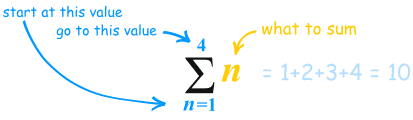# Revision Notes: Sequence Notes | Study Algebra for IIT JAM Mathematics - Mathematics

## Mathematics: Revision Notes: Sequence Notes | Study Algebra for IIT JAM Mathematics - Mathematics

The document Revision Notes: Sequence Notes | Study Algebra for IIT JAM Mathematics - Mathematics is a part of the Mathematics Course Algebra for IIT JAM Mathematics.
All you need of Mathematics at this link: Mathematics

What do we mean by a sequence?
A sequence is basically a succession of numbers which is formed according to some definite rule. The most important thing in the concept of a sequence is that the domain is the set of natural numbers. Sequences may be finite or infinite depending on the number of terms they contain.
If the range of the sequence is the set of real numbers then the sequence is said to be real valued. Similarly if the range is of complex numbers then the sequence is said to be complex valued.
Eg: 1, 3, 5, 7 is a sequence which is formed by addition of 2 to every preceding term.

What is a progression?
If the terms of a sequence are written under some specific condition then the sequence is called a progression.

What is a series?
Series are formed with the terms of the sequence itself. For a given sequence {fn}, the corresponding series is given by f1 + f2 + …. + fn.
Eg: For the sequence 1, 3, 5, 7, …. , the corresponding series is given by
1+3+5+7+……. .
A series is generally expressed using the summation sign like the series (1+3+5+7) can be expressed as Σ (2n-1), where summation runs from 1 to 4.Types of Progressions
1. Arithmetic Progression Basically there are three types of progressions:
2. Geometric Progression
3. Harmonic Progression

All these progressions have been discussed in detail in the coming sections. Here we shall just discuss these topics without going into the intricacies:

Arithmetic Progression
If the first term of the Arithmetic Progression which is generally called as A.P. is ‘a’ and the common difference is ‘d’ then the nth term is given by tn = a+(n-1)d.A sequence of numbers <an> is said to be in arithmetic progression if the difference between the terms is constant i.e. tn – tn-1 is a constant for all n ∈ N. the constant difference between the terms is called the common difference and is denoted by‘d’.
Similarly, the sum of first ‘n’ terms of the A.P. is given by
Sn = n/2 [2a + (n-1) d]
= n/2 [a + l], where l is the last term of the A.P.

Geometric Progression
If the first term of the geometric progression is ‘a’ and the common difference is ‘r’ then the nth term is given by tn = arn-1If every successive term is a fixed multiple of the preceding term then the series is said to be a geometric series. A progression of the form a, ar, ar2, ….. is a geometric progression where the common multiple r is called the fixed ratio. it follows from the definition itself that no term of the G.P can be zero because in such a case the series will get reduced to a zero series.
The sum Sn of the first ‘n’ terms of the GP is given by
Sn = a(rn -1)/ (r-1) , if r ≠1
= na, if r =1.
1 + x + x2 +…. + = 1/(1-x)In case of an infinite G.P. the sum is given by

Harmonic Progression
The nth term of H.P. is given by the sequence a1, a2, …. an, where ai ≠ 0 for every i is said to be in harmonic progression (H.P.) if the sequence 1/a1, 1/a2, …. ,1/an is in A.P.
an =1/[a+(n-1)d], where a = 1/a1 and d = 1/a2 -1/a1.
For harmonic progression problems and solutions, please refer the following sections.
Progressions and Series is important from the perspective of scoring high in IIT JEE as it fetches 1-2 questions in most of the engineering examinations. There are few fixed pattern questions that are generally framed on this topic. You are expected to do all the questions based on this to remain competitive in IIT JEE examination.

The document Revision Notes: Sequence Notes | Study Algebra for IIT JAM Mathematics - Mathematics is a part of the Mathematics Course Algebra for IIT JAM Mathematics.
All you need of Mathematics at this link: MathematicsUse Code STAYHOME200 and get INR 200 additional OFF

## Algebra for IIT JAM Mathematics

70 videos|58 docs

Track your progress, build streaks, highlight & save important lessons and more!

,

,

,

,

,

,

,

,

,

,

,

,

,

,

,

,

,

,

,

,

,

;